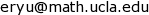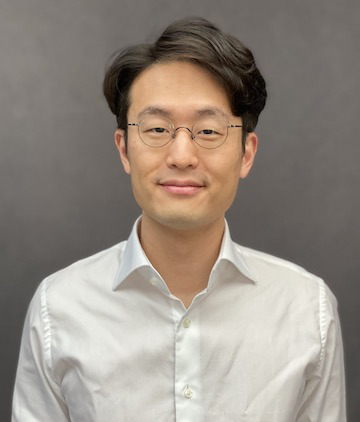## Mathematical Algorithm II, Fall 2022

This is the public course website for Mathematical algorithms II (수학적 알고리즘 2), M1407.000500, Fall 2022. We will use eTL as a secondary website.

## Homework

This class will have irregular homework assignments. Submit completed assignments through eTL.

## Lecture Plans

This course will cover the three separate topics listed below. Each Friday's class will consist of two 75-minute lectures, and each topic will have roughly eight lectures. The two lectures given on the same day will be on different topics, which necessarily means that the presentation of the topics will not be contiguous.

Mathematical rigor will not be a priority for this course. For neural differential equations and diffusion models, we will focus on calculations and derivations while skipping over technical details. For deep reinforcement learning and natural language processing, we should be able to properly derive the key mathematical results.

Neural differential equations and diffusion models:

• Neural ODE
• Continuous-depth normalizing flows
• Stochastic differential equations and Itô calculus
• Fokker–Planck equation and reverse-time diffusion
• Diffusion generative models via stochastic differential equations
• Score matching and discrete-time diffusion models
• Diffusion Schrödinger bridge

Deep reinforcement learning:

• Markov decision process, dynamic programming, value iteration, and Q-value iteration
• Proximal policy optimization
• Deep Q-learning
• Rainbow DQN
• Maximum entropy RL and soft actor-critic

Natural language processing and transformers:

• Sequence models and text preprocessing
• Recurrent neural networks, GRU, and LSTM
• Bidirectional RNN
• Encoder-decoder architecture and machine translation
• Bahdanau attention
• Vision transformers

## Course Information

### Instructor

Ernest K. Ryu, 27-205,### Lectures

Fridays 2:00–5:50pm at 24-101. Lectures will be mostly based on slides. In-person attendance is required.

### Exams

This class will have a final exam. (No midterm.) This exam is open-book in the sense that you may use any non-electronic resource.

• Final exam: Final exam: 12/22, 1:00–5:00pm, location TBD.